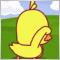Looking for: RSI value from several timeframe in one oscillator79

Hi everyone.

I am looking for custom RSI indicator that calculate the value from several timeframe and represent it into an RSI oscillator or maybe displaying its value on the chart in number form.

Here's how it works.

1. Assume I am trading in m15 timeframe.

2. This custom RSI will take current RSI value in 3 different timeframe and sum it all up and divide it by 3 to give an average.
In this case, I am using m15, h1 and h4.

3. So this average value will be represented on the m15 timeframe as a custom RSI or maybe just display the current average value in number on the chart.

So, is this custom RSI is possible to be coded?

If yes, can someone code it for me and share it here?